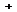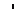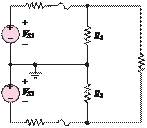### Create an Account

Already have account?

### Forgot Your Password ?

Home / Questions / In the circuit shown in Figure P3 33 F1 and F2 are fuses Under normal conditions they are ...

# In the circuit shown in Figure P3 33 F1 and F2 are fuses Under normal conditions they are modeled as a short circuit However if excess current flows through a fuse its element melts and the fuse

In the circuit shown in Figure P3.33, F1 and F2 are fuses. Under normal conditions they are modeled as a short circuit. However, if excess current flows through a fuse, its element melts and the fuse “blows” (i.e., it becomes an open circuit).

VS1 = VS2 = 115 V

respect to

v1

+VS1

1

 v

 .

n

VS2

Rw1

v2     Rw2

1        2R1

R2

v&#39;n

3

v1&#39;

R1  = R2  = 5 Q      R3  = 10 Q

R4 = R5 = 200 mQ

Normally, the voltages across R1, R2, and R3 are 106.5,

−106.5, and 213.0 V. If F1 now blows, or opens, determine, using KCL and node analysis, the new

+       –+

VS3

v3

Rw3

v&#39;2

R3

v3&#39;

voltages across R1, R2, and R3.R4          F1

Jun 19 2020 View more View Less

#### Answer (Solved)Subscribe To Get Solution